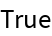#Function Repository Resource:

# ReferenceAngleDegree

Compute the reference angle to a given input angle using degrees

Contributed by: Paco Jain (Wolfram Research)
 ResourceFunction["ReferenceAngleDegree"][θ] gives the reference angle to the angle θ in degrees.

## Details and Options

ResourceFunction["ReferenceAngleDegree"] takes all angles to be measured in degrees.
The reference angle to a given input angle θ is defined geometrically as follows: First, start with a ray having its tail at the origin and pointing along the positive x axis. Next, rotate this ray counterclockwise about the origin by the angle θ. Finally, the reference angle to θ is then the smallest positive angle that this ray makes with the x axis (either positive or negative).
The reference angle of θ in terms of θ can be found via the formula 90-|mod(θ,180)-90|.

## Examples

### Basic Examples

Compute a reference angle:

 In:=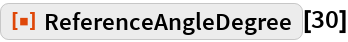Out=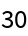Compute the reference angle for angles lying in each of the four quadrants:

 In:=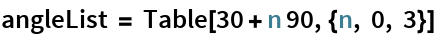Out=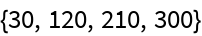In:=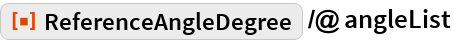Out=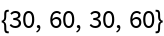Plot the reference angle function for all angles between 0 and 360 degrees:

 In:=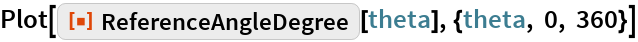Out=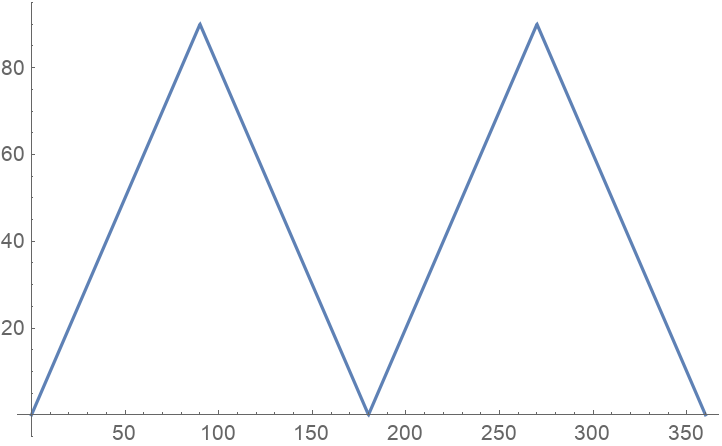### Scope

ReferenceAngleDegree works directly on Quantity expressions with units of "Radians":

 In:=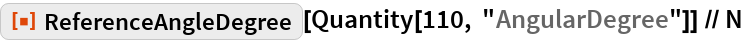Out=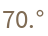ReferenceAngleDegree works directly on DMS lists of the type returned by DMSList:

 In:=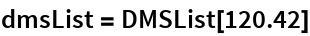Out=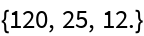In:=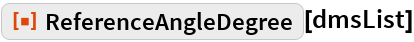Out=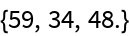ReferenceAngleDegree works directly on DMS strings of the type returned by DMSString:

 In:=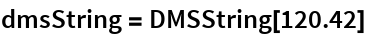Out=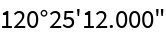In:=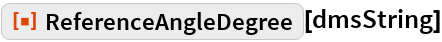Out=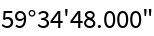### Properties and Relations

ReferenceAngleDegree will remain unevaluated for non-numeric input:

 In:=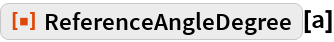Out=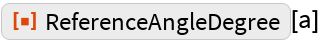ReferenceAngleDegree throws away the sign of its input:

 In:=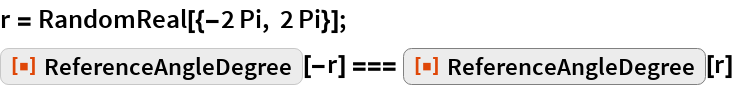Out=